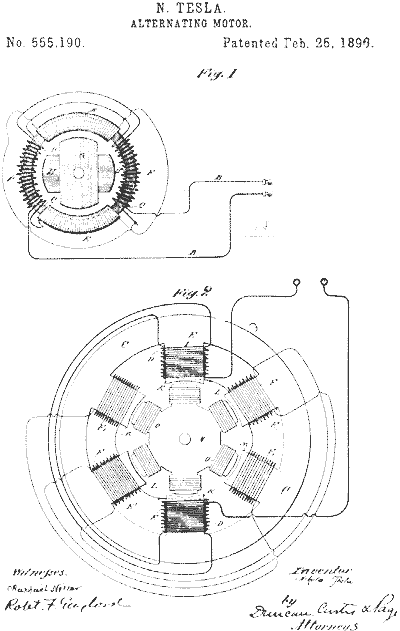top of pageI have been exploring Nikola Tesla's Hexagram and the 3,6,9 ratio. By connecting each end point of the outside angles, then bisecting them.

After this, I created an action in Photoshop that would replicate the object, then rotate it 1 degree, until it completes a 360 degree rotation -1.

Tesla was into Numerology and was obsessed with the numbers 3, 6, and 9.

Each bisected angle x the number of angles then broken down, all equal 9. (i.e., 3 + 6 + 9 = 18 and 1 + 8 = 9)

What you will see in the animation is a vortex that appears.

Something Tesla was working on was vortex electricity, and free energy. Is this a visual representation of what Tesla was working on?

Another thought, if this is a cross section of a tower he built in NY 120 feet underground and extending above ground, it might be the correct shape to harness electricity from a magnetic field created by the earth. As long as each tower was placed on the appropriate grid following the magnetic field mapped over the earth on strategic points on the globe.

(Another example might be the Pyramids in each of the  continents).

bottom of page